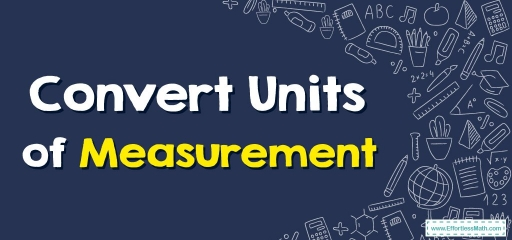# Convert Units of Measurement

In this article, we want to know more about units of measurement and how to convert them to each other.Before measuring anything, you must understand the correct unit to apply.

Dimensional analysis is the method of converting between units.

The International System of Units (SI) stipulates a set of $$7$$ base units by which all additional units of measurement get created. The units derived are based on these $$7$$ base units.

## Related Topics

The $$7$$ base $$SI$$ units are as follows: [Physical Quantity: unit symbol (unit’s name)]

• Length: m (meter)
• Mass: kg (kilogram)
• Time: s (second)
• Electric Current: A (Ampere)
• Thermodynamic Temperature: K (degrees Kelvin)
• Quantity of Substance: mol (mole)
• Luminous Intensity: cd (candela)

The unit analysis is a kind of proportional reasoning in which an offered measurement can be multiplied via a recognized proportion or ratio to provide an answer that has a different dimension or unit.

## Measurement Conversions

Whenever we must compare values for the provided quantities or do math operations on these, we must have a common unit of measurement. For instance, we can add together $$13$$ meters and $$11$$ meters and end up with $$24$$ meters, but you can’t add up $$13$$ meters to $$11$$ inches. With those, we must know and utilize ‘measurement conversion’ to be able to convert provided units into some sort of common units. No matter if it’s mass, length, time, or temperature, there’s a formula to utilize for getting the values for any conversion. Provided here is a measurement chart you can utilize for these types of conversions.

Unit conversion is a procedure that has several steps involving multiplying or dividing via a mathematical factor or, specifically a conversion factor. This procedure could additionally need you to select the right amount of important digits, and use rounding. We use different units of conversion to measure different kinds of parameters.

## Converting bigger units to lesser units

To convert a bigger unit to a lesser unit (like millimeters to centimeters), firstly see the amount of lesser units you need to make one needed to make $$1$$ larger unit. Then, multiply that number by the number of larger units.

## Converting smaller units to larger units

To convert a lesser unit to a bigger unit (like centimeters to millimeters, you must divide it by the amount of lesser units required to make one bigger unit.

### Convert Units of Measurement – Example 1:

Convert to an appropriate measurement unit. (Round to the nearest Hundredths)

$$12$$ inches$$=$$___cm

Solution:

We know that $$1$$ inch $$= 2.54$$ cm. So, to convert inch to cm we should multiply $$12$$ by $$2.54$$. Therefore: $$12× 2.54 =30.48$$. The answer is $$30.48$$ cm

### Convert Units of Measurement – Example 2:

Convert to an appropriate measurement unit.

$$4$$ mile$$=$$____ yard

Solution:

We know that $$1$$ mile $$= 1760$$ yards. So, to convert mile to yard we should multiply $$4$$ by $$1760$$. Therefore: $$4× 1760 =7,040$$. The answer is $$7,040$$ yard

## Exercises for Convert Units of Measurement

### Convert to an appropriate measurement unit. (Round to the nearest Hundredths)

1. $$\color{blue}{25cm=?m}$$
2. $$\color{blue}{20feet=?inches}$$
3. $$\color{blue}{5yards=?inches}$$
4. $$\color{blue}{50feet=?m}$$
1. $$\color{blue}{0.25}$$
2. $$\color{blue}{240}$$
3. $$\color{blue}{180}$$
4. $$\color{blue}{15.24}$$

### What people say about "Convert Units of Measurement - Effortless Math: We Help Students Learn to LOVE Mathematics"?

No one replied yet.

X
30% OFF

Limited time only!

Save Over 30%

SAVE $5 It was$16.99 now it is \$11.99